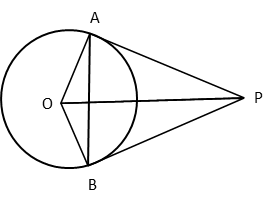# CBSE 2017 Board Paper

Class 10 Math - AI Set 3 - Q6. Geometry

#### Video ExplanationLet ‘O’ be the centre of the circle.
PA and PB are two tangents drawn from point P.
AB is a chord to the circle.
OA and OB are radii to the circle.

∠OAP = ∠OBP = 90o because the angle between the tangent and the radius where the tangent meets the circle is a right angle.

Because OA and OB are radii to the circle, triangle AOB is isosceles.
Therefore, ∠OAB = ∠OBA.

Let the measures of ∠OAB = ∠OBA be θ.
∠PAB = ∠OAP - ∠OAB = 90 – θ
∠PBA = ∠OBP - ∠OBA = 90 – θ
i.e., ∠PAB = ∠PBA
The tangents PA and PB make equal angles with the chord AB.

.